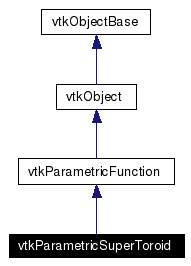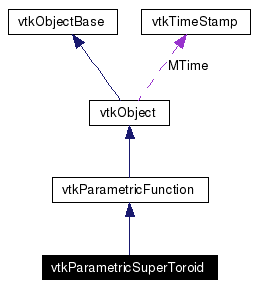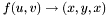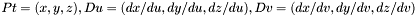# vtkParametricSuperToroid Class Reference

#include <vtkParametricSuperToroid.h>

Inheritance diagram for vtkParametricSuperToroid:[legend]
Collaboration diagram for vtkParametricSuperToroid:[legend]
List of all members.

## Detailed Description

Generate a supertoroid.

vtkParametricSuperToroid generates a supertoroid. Essentially a supertoroid is a torus with the sine and cosine terms raised to a power. A supertoroid is a versatile primitive that is controlled by four parameters r0, r1, n1 and n2. r0, r1 determine the type of torus whilst the value of n1 determines the shape of the torus ring and n2 determines the shape of the cross section of the ring. It is the different values of these powers which give rise to a family of 3D shapes that are all basically toroidal in shape.

For further information about this surface, please consult the technical description "Parametric surfaces" in http://www.vtk.org/documents.php in the "VTK Technical Documents" section in the VTk.org web pages.

Warning:
Care needs to be taken specifying the bounds correctly. You may need to carefully adjust MinimumU, MinimumV, MaximumU, MaximumV.
Thanks:
Andrew Maclean a.maclean@cas.edu.au for creating and contributing the class.
Tests:
vtkParametricSuperToroid (Tests)

Definition at line 51 of file vtkParametricSuperToroid.h.

## Public Types

typedef vtkParametricFunction Superclass

## Public Member Functions

virtual const char * GetClassName ()
virtual int IsA (const char *type)
void PrintSelf (ostream &os, vtkIndent indent)
virtual void Evaluate (double uvw, double Pt, double Duvw)
virtual double EvaluateScalar (double uvw, double Pt, double Duvw)
virtual int GetDimension ()
virtual void SetRingRadius (double)
virtual double GetRingRadius ()
virtual void SetCrossSectionRadius (double)
virtual double GetCrossSectionRadius ()
virtual void SetXRadius (double)
virtual double GetXRadius ()
virtual void SetYRadius (double)
virtual double GetYRadius ()
virtual void SetZRadius (double)
virtual double GetZRadius ()
virtual void SetN1 (double)
virtual double GetN1 ()
virtual void SetN2 (double)
virtual double GetN2 ()

## Static Public Member Functions

static int IsTypeOf (const char *type)
static vtkParametricSuperToroidSafeDownCast (vtkObject *o)
static vtkParametricSuperToroidNew ()

## Protected Member Functions

vtkParametricSuperToroid ()
~vtkParametricSuperToroid ()

## Protected Attributes

double RingRadius
double CrossSectionRadius
double XRadius
double YRadius
double ZRadius
double N1
double N2

## Member Typedef Documentation

 Reimplemented from vtkParametricFunction. Definition at line 54 of file vtkParametricSuperToroid.h.

## Constructor & Destructor Documentation

 vtkParametricSuperToroid::vtkParametricSuperToroid ( )  [protected]

 vtkParametricSuperToroid::~vtkParametricSuperToroid ( )  [protected]

## Member Function Documentation

 virtual const char* vtkParametricSuperToroid::GetClassName ( )  [virtual]
 Reimplemented from vtkParametricFunction.

 static int vtkParametricSuperToroid::IsTypeOf ( const char * type )  [static]
 Return 1 if this class type is the same type of (or a subclass of) the named class. Returns 0 otherwise. This method works in combination with vtkTypeRevisionMacro found in vtkSetGet.h. Reimplemented from vtkParametricFunction.

 virtual int vtkParametricSuperToroid::IsA ( const char * type )  [virtual]
 Return 1 if this class is the same type of (or a subclass of) the named class. Returns 0 otherwise. This method works in combination with vtkTypeRevisionMacro found in vtkSetGet.h. Reimplemented from vtkParametricFunction.

 static vtkParametricSuperToroid* vtkParametricSuperToroid::SafeDownCast ( vtkObject * o )  [static]
 Reimplemented from vtkParametricFunction.

 void vtkParametricSuperToroid::PrintSelf ( ostream & os, vtkIndent indent )  [virtual]
 Methods invoked by print to print information about the object including superclasses. Typically not called by the user (use Print() instead) but used in the hierarchical print process to combine the output of several classes. Reimplemented from vtkParametricFunction.

 static vtkParametricSuperToroid* vtkParametricSuperToroid::New ( )  [static]
 Construct a supertoroid with the following parameters: MinimumU = 0, MaximumU = 2*Pi, MinimumV = 0, MaximumV = 2*Pi, JoinU = 1, JoinV = 1, TwistU = 0, TwistV = 0, ClockwiseOrdering = 1, DerivativesAvailable = 0, RingRadius = 1, CrossSectionRadius = 0.5, N1 = 1, N2 = 1, XRadius = 1, YRadius = 1, ZRadius = 1, a torus in this case. Reimplemented from vtkObject.

 virtual int vtkParametricSuperToroid::GetDimension ( )  [inline, virtual]
 Return the parametric dimension of the class. Implements vtkParametricFunction. Definition at line 65 of file vtkParametricSuperToroid.h.

 virtual void vtkParametricSuperToroid::SetRingRadius ( double )  [virtual]
 Set/Get the radius from the center to the middle of the ring of the supertoroid. Default = 1.

 virtual double vtkParametricSuperToroid::GetRingRadius ( )  [virtual]
 Return the parametric dimension of the class.

 virtual void vtkParametricSuperToroid::SetCrossSectionRadius ( double )  [virtual]
 Set/Get the radius of the cross section of ring of the supertoroid. Default = 0.5.

 virtual double vtkParametricSuperToroid::GetCrossSectionRadius ( )  [virtual]
 Set/Get the radius of the cross section of ring of the supertoroid. Default = 0.5.

 virtual void vtkParametricSuperToroid::SetXRadius ( double )  [virtual]
 Set/Get the scaling factor for the x-axis. Default = 1.

 virtual double vtkParametricSuperToroid::GetXRadius ( )  [virtual]
 Set/Get the scaling factor for the x-axis. Default = 1.

 virtual void vtkParametricSuperToroid::SetYRadius ( double )  [virtual]
 Set/Get the scaling factor for the y-axis. Default = 1.

 virtual double vtkParametricSuperToroid::GetYRadius ( )  [virtual]
 Set/Get the scaling factor for the y-axis. Default = 1.

 virtual void vtkParametricSuperToroid::SetZRadius ( double )  [virtual]
 Set/Get the scaling factor for the z-axis. Default = 1.

 virtual double vtkParametricSuperToroid::GetZRadius ( )  [virtual]
 Set/Get the scaling factor for the z-axis. Default = 1.

 virtual void vtkParametricSuperToroid::SetN1 ( double )  [virtual]
 Set/Get the shape of the torus ring. Default = 1.

 virtual double vtkParametricSuperToroid::GetN1 ( )  [virtual]
 Set/Get the shape of the torus ring. Default = 1.

 virtual void vtkParametricSuperToroid::SetN2 ( double )  [virtual]
 Set/Get the shape of the cross section of the ring. Default = 1.

 virtual double vtkParametricSuperToroid::GetN2 ( )  [virtual]
 Set/Get the shape of the cross section of the ring. Default = 1.

 virtual void vtkParametricSuperToroid::Evaluate ( double uvw, double Pt, double Duvw )  [virtual]
 A supertoroid. This function performs the mapping, returning it as Pt. It also returns the partial derivatives Du and Dv.. Then the normal is. Implements vtkParametricFunction.

 virtual double vtkParametricSuperToroid::EvaluateScalar ( double uvw, double Pt, double Duvw )  [virtual]
 Calculate a user defined scalar using one or all of uvw, Pt, Duvw. uvw are the parameters with Pt being the the cartesian point, Duvw are the derivatives of this point with respect to u, v and w. Pt, Duvw are obtained from Evaluate(). This function is only called if the ScalarMode has the value vtkParametricFunctionSource::SCALAR_FUNCTION_DEFINED If the user does not need to calculate a scalar, then the instantiated function should return zero. Implements vtkParametricFunction.

## Member Data Documentation

 double vtkParametricSuperToroid::RingRadius [protected]
 Definition at line 133 of file vtkParametricSuperToroid.h.

 double vtkParametricSuperToroid::CrossSectionRadius [protected]
 Definition at line 134 of file vtkParametricSuperToroid.h.

 double vtkParametricSuperToroid::XRadius [protected]
 Definition at line 135 of file vtkParametricSuperToroid.h.

 double vtkParametricSuperToroid::YRadius [protected]
 Definition at line 136 of file vtkParametricSuperToroid.h.

 double vtkParametricSuperToroid::ZRadius [protected]
 Definition at line 137 of file vtkParametricSuperToroid.h.

 double vtkParametricSuperToroid::N1 [protected]
 Definition at line 138 of file vtkParametricSuperToroid.h.

 double vtkParametricSuperToroid::N2 [protected]
 Definition at line 139 of file vtkParametricSuperToroid.h.

The documentation for this class was generated from the following file:

Generated on Tue Jan 22 00:06:07 2008 for VTK by1.4.3-20050530